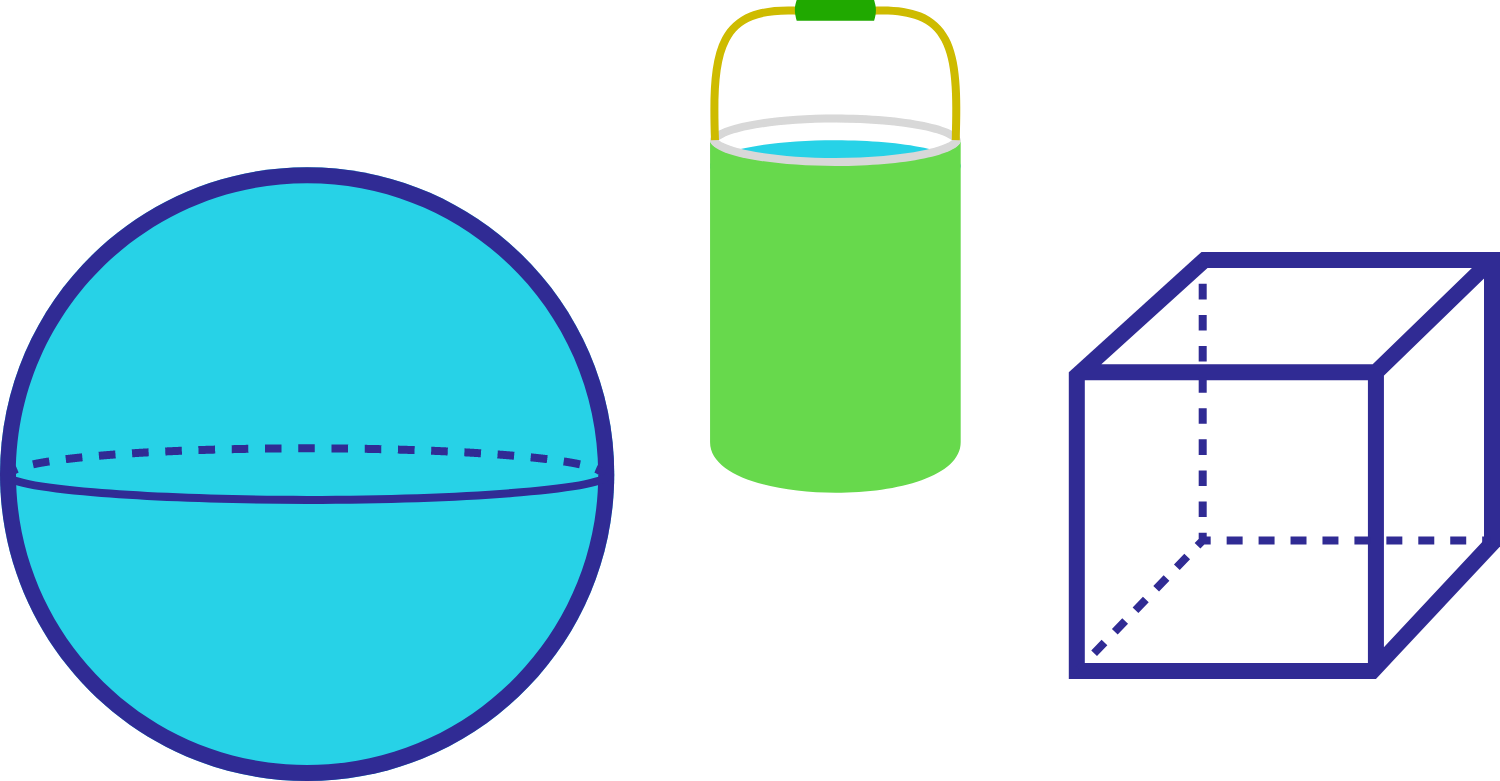# How much paint do you need?

Geometry Level 1You have bought a kind of paint that could paint 50 $\text{m}^{2}$ per quart. Then you used approximately 6.28 quarts of paint to color the surface of a sphere. Now you wish to paint a cube that has a side length the same as the sphere's radius. How much paint do you need, in quarts?

(P.S. use 3.14 as an approximation for pi., and $\text{m}$ means meter.)

×